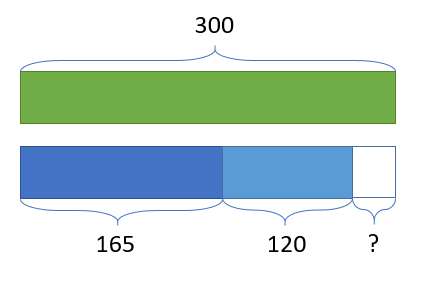Smartick is an advanced online program that teaches kids math and coding in only 15 min. a day

Oct16

# Using Singapore Bars to Help Solve Problems II

At Smartick, we place great importance on solving problems. We use a phase model, like in Singapore, and bars for representation. Visualization is fundamental to learning math. The bars help to visualize resolution processes, but they are just one of many tools that we use.

Let’s look at an example of how to apply Singapore bars to solve problems.

### Addition and subtraction problems with Singapore barsThere are 300 spectators at a basketball game. 165 are men, 120 are women, and the rest are children. How many children are there?

First we use a bar to represent the total of spectators watching the match:

Now we represent the number of men and women.The part of the bar we have left represents the number of children. The bars help us to understand the problem visually and find a way to solve it.

We can see that if the bar was whole, it would be equal to the number of men plus the number of women, plus the number of children. To find out the number of children watching the game, we have to perform two subtractions:

300 – 165 – 120 = 15

There are 15 children watching the basketball game.

### Multiplication and division problems with Singapore barsA book costs 30 dollars. If a pen costs 5 times less than the book, how much does the pen cost?

First of all, we use a bar to represent the 30 dollars that the book costs.Since the pen costs 5 times less, we divide the bar into 5 equal parts.

The 5 units represent 30 in total.

To find out what 1 unit costs, we have to divide 30 by 5.

30 ÷ 5 = 6

The pen costs 6 dollars.

So there you have some examples of how we can apply Singapore bars to help us solve problems, look out for more in the future!

If you want to keep learning math, register with Smartick and enjoy a free trial.